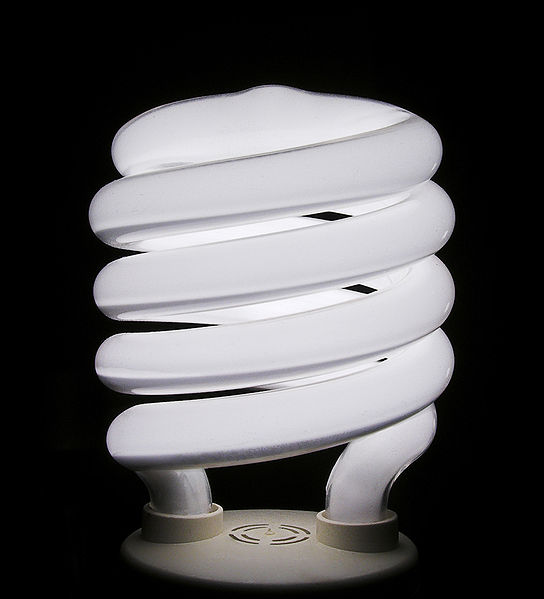## Energy Usage

The energy used is the time integral of the electric power.

### Learning Objectives

Formulate the relationship between the energy usage and the electric power

### Key Takeaways

#### Key Points

• Recall that power is the rate work is done, or the rate at which energy is consumed or produced. In terms of current and voltage it is P=IV.
• The energy used is the amount of charge q moved through voltage V in a time interval t. It is equal to the integral of power over time.
• A common unit used to describe energy usage is the kilowatt-hour, the energy of 1000 W acting over one hour.

#### Key Terms

• kilowatt-hour: a unit of electrical energy equal to that done by one kilowatt acting for one hour; equal to 3.6 mega-joules. Symbol: kWh.

In many cases it is necessary to calculate the energy usage by an electric device or a collection of devices, such as in a home. For example, we (or the electric power utility) may want to calculate the amount of money owed for electricity consumed. In another case, we might need to determine the energy necessary to power a component or apparatus for a given period of time. The last distinction is crucial – the energy used by a circuit or component is the time integral of the electric power.

### Power

Recall that power is the rate at which work is done – or the rate at which energy is consumed or produced – and is measured in watts (W). The electric power in watts produced by an electric current I consisting of a charge of Q coulombs every t seconds passing through an electric potential (voltage) difference of V is $\text{P} = \frac{\text{QV}}{\text{t}} = \text{IV}$, where Q is electric charge in coulombs, t is time in seconds, I is electric current in amperes, and V is electric potential or voltage in volts.

In resistive circuits where Ohm ‘s Law applies, the power can be expressed as $\text{P} = \text{I}^2\text{R} = \frac{\text{V}^2}{\text{R}}$, where R is the electrical resistance. Power isn’t necessarily constant; it may vary over time. The general expression for electric power is then

$\text{P}(\text{t})=\text{I}(\text{t})\text{V}(\text{t})$

where the current I and voltage V may be time variable.

### Energy

In any given time interval, the energy consumed (or provided, depending on your perspective) is given by $\text{PE}=\text{qV}$, where E is the electric energy, V is the voltage, and q is the amount of charge moved in the time interval under consideration. We can relate the total energy consumed to the power by integrating over time:. Positive energy corresponds to consumed energy and negative energy corresponds to energy production. Note that a circuit element having a power profile that is both positive and negative over some time interval could consume or produce energy according to the sign of the integral of power. If the power is constant over the time interval then the energy can be expressed simply as:

$\text{E}=\text{Pt}$.

### Units of Energy Usage

We are of course very familiar with the SI unit of energy, the joule. However, typically, residential energy bills state household energy consumption in kilowatt-hours (kWh). Additionally, this unit is often seen elsewhere when the energy usage of power consuming devices, structures, or jurisdictions is under consideration. We can parse out the conversion from kilowatt-hours to joules in this way: 1 W = 1 J/s and a kilowatt is 1000 W while one hour is 3,600 seconds, so 1 kWh is (1000 J/s)(3600 s)=3,600,000 joules. This is the scale of American home energy usage, which is on the order of hundreds of kilowatt-hours per month.

### Reducing Energy Usage

The electrical energy (E) used can be reduced either by reducing the time of use or by reducing the power consumption of that appliance or fixture. This will not only reduce the cost, but it will also result in a reduced impact on the environment. Improvements to lighting are some of the fastest ways to reduce the electrical energy used in a home or business. About 20% of a home’s use of energy goes to lighting, while the number for commercial establishments is closer to 40%. Fluorescent lights are about four times more efficient than incandescent lights—this is true for both the long tubes and the compact fluorescent lights (CFL). Thus, a 60-W incandescent bulb can be replaced by a 15-W CFL, which has the same brightness and color. CFLs have a bent tube inside a globe or a spiral-shaped tube, all connected to a standard screw-in base that fits standard incandescent light sockets. (Original problems with color, flicker, shape, and high initial investment for CFLs have been addressed in recent years. ) The heat transfer from these CFLs is less, and they last up to 10 times longer.Compact Fluorescent Light (CFL): CFLs are much more efficient than incandescent bulbs and so consume much less energy for the intensity light produces.